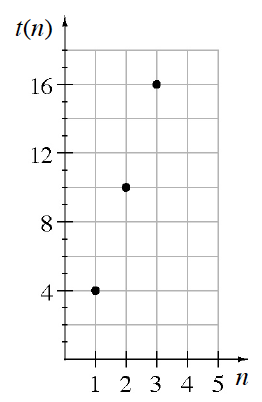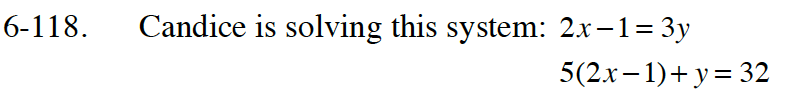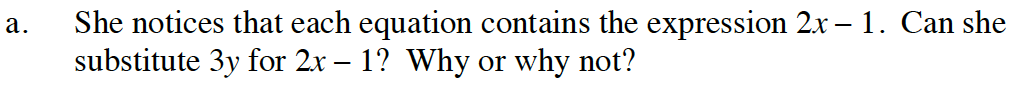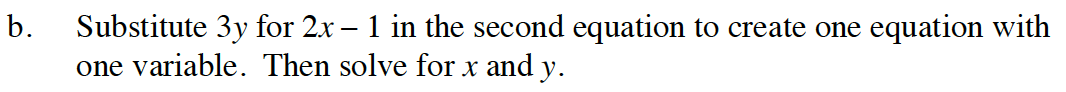Home > GB8I > Chapter cc46 > Lesson cc46.4.1 > Problem6-118

6-118.
1. Candice is solving this system: 2x − 1 = 3y Homework Help ✎
5(2x − 1) + y = 32

1. She notices that each equation contains the expression 2x − 1. Can she substitute 3y for 2x − 1? Why or why not?

2. Substitute 3y for 2x − 1 in the second equation to create one equation with one variable. Then solve for x and y.Try it for yourself. Does it work?